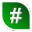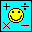### Windows / Mathematics#### Popularity

Free

ODEcalc (tm): Ordinary Differential Equation (ODE) Calculator! Solves ODEs

Mathematics | Windows | 15 June 2015#### Popularity

Mathematics | Windows |#### Popularity

Represents prisms and pyramids in 3D space. Supports interactive 3D animation.

Mathematics | Windows | 20 September 2004#### Popularity

Mathematics | Windows |#### Popularity

Mathematics | Windows |#### Popularity

Mathematics | Windows |#### Popularity

Interactive software that helps students understand and work with fractions.

Mathematics | Windows | 15 April 2004#### Popularity

Generate random whole and floating point numbers within specified range

Mathematics | Windows | 31 December 2017#### Popularity

Learn, practice, and test the mathematical order of operations.

Mathematics | Windows | 30 September 2006#### Popularity

Free

ODEcalc (tm): Ordinary Differential Equation (ODE) Calculator! Solves ODEs

Mathematics | Windows | 15 June 2015